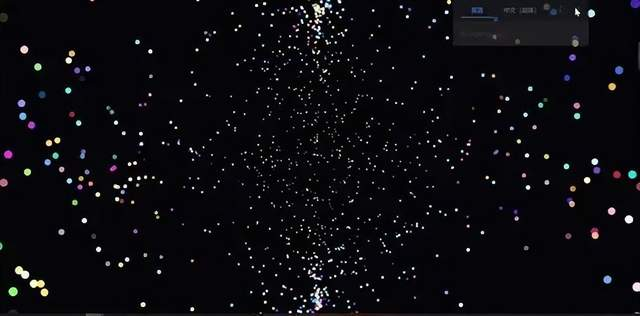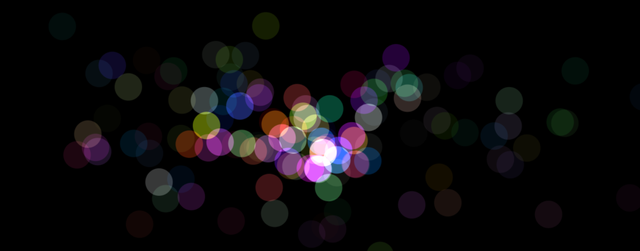# Canvas之鼠标滑动特效# 什么是 Canvas

<canvas> 是 HTML5 新增的元素，可用于通过使用 JavaScript 中的脚本来绘制图形。例如，它可以用于绘制图形、制作照片、创建动画，甚至可以进行实时视频处理或渲染。

<canvas> 标签允许脚本语言动态渲染位图像。<canvas> 标签创建出了一个可绘制区域，JavaScript 代码可以通过一套完整的绘图功能类似于其他通用二维的 API 访问该区域，从而生成动态的图形。

# 案例-鼠标滑动效果# 页面搭建

``````<!DOCTYPE html>
<html lang="en">

<meta charset="UTF-8">
<meta name="viewport" content="width=device-width, initial-scale=1.0">
<meta http-equiv="X-UA-Compatible" content="ie=edge">
<title>Document</title>

<body>
<canvas id="myCanvas"></canvas>
</body>

</html>
``````

``````* {
margin: 0;
}

body {
overflow: hidden;
}

#myCanvas {
background-color: #000; /* 黑色 */
}
``````

# 逻辑交互

``````var myCanvas = document.getElementById('myCanvas');
var ctx = myCanvas.getContext("2d");
var starlist = [];
function init() {
// 设置canvas区域的范围为整个页面
myCanvas.width = window.innerWidth;
myCanvas.height = window.innerHeight;
};
init();
``````

``````window.onresize = init; // 监听屏幕大小改变 重新为canvas大小赋值
``````

``````// 当鼠标移动时 将鼠标坐标传入构造函数 同时创建一个对象
// 将对象push到数组中，画出来的彩色小点可以看作每一个对象中记录着信息 然后存在数组中
starlist.push(new Star(e.offsetX, e.offsetY));
});
``````

``````// 随机函数封装,设置坐标
function random(min, max) {
// 设置生成随机数公式
return Math.floor((max - min) * Math.random() + min);
};
``````

``````// 定义了一个构造函数进行对象构造
function Star(x, y) {
// 将坐标存在每一个点的对象中
this.x = x;
this.y = y;
// 设置随机偏移量
this.vx = (Math.random() - 0.5) * 3;
this.vy = (Math.random() - 0.5) * 3;
this.color = 'rgb(' + random(0, 256) + ',' + random(0, 256) + ',' + random(0, 256) + ')';
this.a = 1; // 初始透明度
this.draw(); // 把对象绘制到页面
}
``````

``````//star对象原型上封装方法
Star.prototype = {
// canvas根据数组中存在的每一个对象的小点信息开始画
draw: function () {
ctx.beginPath();
ctx.fillStyle = this.color;
// 图像覆盖  显示方式 lighter 会将覆盖部分的颜色重叠显示出来
ctx.globalCompositeOperation = 'lighter'
ctx.globalAlpha = this.a;
ctx.arc(this.x, this.y, 30, 0, Math.PI * 2, false);
ctx.fill();
this.updata();
},
updata() {
// 根据偏移量更新每一个小点的位置
this.x += this.vx;
this.y += this.vy;
// 透明度越来越小
this.a *= 0.98;
}
}

``````

``````// 将小球渲染到页面上
function render() {
// 每一次根据改变后数组中的元素进行画圆圈  把原来的内容区域清除掉
ctx.clearRect(0, 0, myCanvas.width, myCanvas.height)

// 根据存在数组中的每一位对象中的信息画圆
starlist.forEach(function (ele, i) {
ele.draw();
// 如果数组中存在透明度小的对象 ，给他去掉 效果展示逐渐消失
if (ele.a < 0.05) {
starlist.splice(i, 1);
}
});
requestAnimationFrame(render);
}
render();
``````